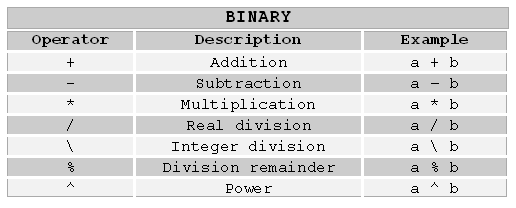## 9.2 Arithmetic operators

Hollywood supports the following arithmetic operators:It should be pretty self-explaining how to use these operators so here is only a brief description of every operator:

Adds a and b, e.g. 5 + 3 = 8

Subtraction: a - b
Subtracts b from a, e.g. 10 - 5 = 5

Multiplication: a * b
Multiplies a by b, e.g. 10 * 8 = 80

Real division: a / b
Does an exact division. Result might be a floating point value, e.g. 5 / 2 = 2.5. b must not be 0

Integer division: a \ b
Divides a by b. Decimal places will be deleted, e.g. 5 \ 2 = 2 b must not be 0

Division remainder: a % b
Returns the integer remainder of the division a \ b, e.g. 5 % 2 = 1 b must not be 0

Power: a ^ b
Calculates a to the power of b, e.g. 2 ^ 8 = 256

Negation: -a
Negates a, e.g. --5 = 5

Show TOC